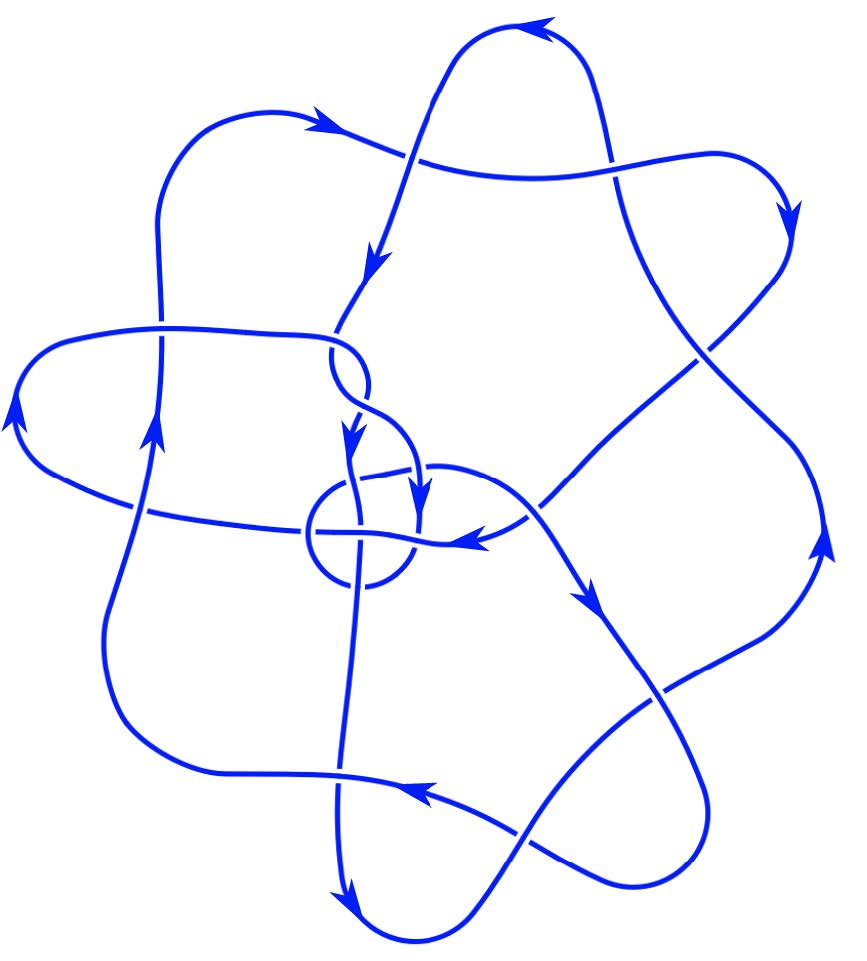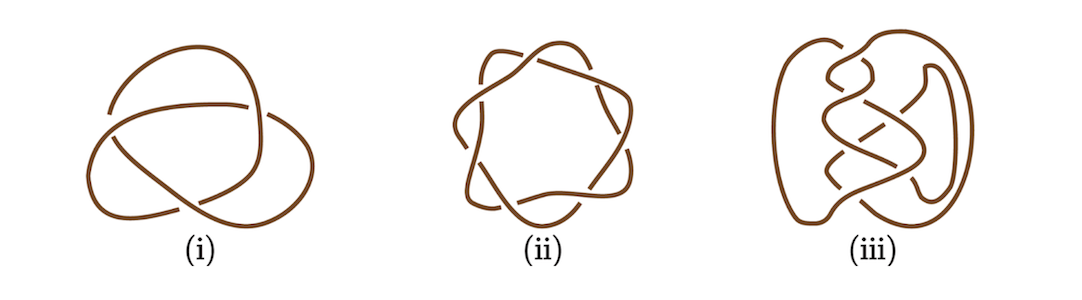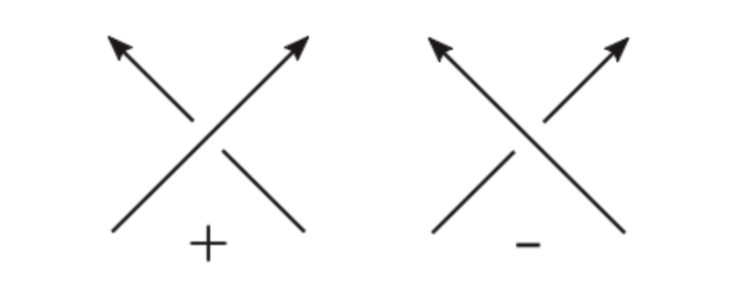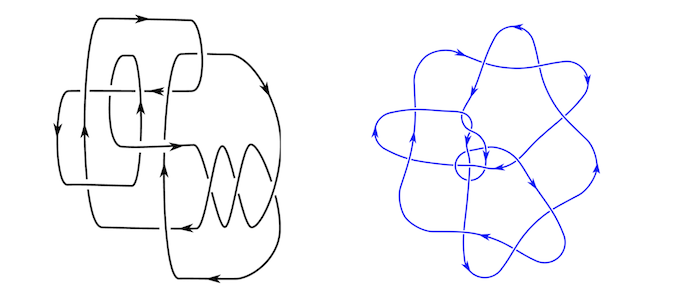### Highlights#### Khovanov Homology of Positive Knots

ICERM - May 2023(based on joint work with Naageswaran Manikandan and Leo Mousseau [KMMS23])

Knot theory is the branch of mathematics studying mathematical knots. One can create a physical model of a knot by taking a rope, tangling it in space, and then gluing its ends together. Some examples of knots are shown in Figures 1 and 3.Figure 1. Three different views on the trefoil knot, the simplest non-trivial knot.We say that two knots K₁ and K₂ are equivalent if K₁ can be deformed continuously (without cutting the rope) into K₂. The three knots in Figure 1 are all equivalent. In the more precise language of differential topology, we say that a knot is the isotopy class of a smooth embedding of the circle into the 3-sphere.

Often knots are studied via so-called knot diagrams, generic projections to 2-planes with stored over and under information at each crossing, as already done silently in Figure 1.

Diagrams (and therefore knots) can be oriented by choosing one of the two possible directions when traveling through it. Each crossing in an oriented diagram can be classified into positive or negative, according to the convention shown in Figure 2. An interesting subclass of knots is given by so-called positive knots. A knot is positive if it admits a diagram where all crossings are positive.Figure 2. We can assign to every crossing a sign.Since the diagrams of the trefoil shown in Figure 1(i) and the diagram of the 12-crossing knot K12n110 in Figure 3(i) are readily seen to have only positive crossings (with any of the two possible orientations), we know both represent positive knots. On the other hand, we observe that the other knot diagrams of the trefoils in Figure 1 are not positive. Generalizing this example, we see that every positive knot also has non-positive diagrams. This makes it hard to answer if a given knot is positive. For example, the diagram of the knot o9_34097 shown in Figure 3 contains negative crossings. Since there could be a potentially different diagram of o9_34097 that is positive, it was not known if o9_34097 is positive.Figure 3. Knot diagrams of two more complicated knots, created using KLO [Swe]. The knot on the left is indexed by K12n110, where the 12 means that it has no diagram with less than 12 crossings. The knot on the right is the census knot o9_34097. Here o9 indicates that the complement of it admits an ideal triangulation with 9 tetrahedra [Dun20].While there exists a theoretical algorithm that decides in finite time if a given knot is positive or not, this algorithm has a factorial run time and is thus a purely theoretical result that is not working in practice even for very simple knots.

In our project, which can be found at [KMMS23], we have developed a different approach to obstruct positivity of knots. For any knot, Khovanov has developed a computable knot invariant called Khovanov homology, which is given by a doubly graded sequence of abelian groups. We show that some of these Khovanov homology groups take special values for positive knots, extending and generalizing earlier work from [Kho03, PS14, Sto10]. Then we can for example compute the Khovanov homology of o9_34097 and observe that it does not fulfill these properties. Thus we have shown that o9_34097 does not admit any positive diagram.

The same approach works to show that other knots are not positive for which it was previously unknown. Although our obstruction is known to be not perfect, it seems to be strong. Among the 2977 prime knots with at most 12 crossings, exactly 246 are positive and the Khovanov homology obstructs all the other 2731 knots from being positive.

Positive knots are closely related to braids, the topic of the semester program at ICERM (February - May 2022) where this project started [CEE+22].

References
[CEE+22] M. Culler, B. Elias, J. Etnyre, B. Farb, J. González-Meneses, M. Hedden, K. Kawamuro, A. Licata, and J. Licata, Semester program on Braids, Institute for Computational and Experimental Research in Mathematics (ICERM), Providence, Rhode Island (2022).
[Dun20] N. M. Dunfield, A census of exceptional Dehn fillings, Characters in low-dimensional topology, Contemp. Math., vol. 760, Amer. Math. Soc., [Providence], RI,  © 2020, pp. 143–155. MR 4193924
[Kho03] M. Khovanov, Patterns in knot cohomology I, Experiment. Math. 12 (2003), 365–374. MR 2034399
[KMMS23] M. Kegel, N. Manikandan, L. Mousseau, and M. Silvero, Khovanov homology of positive links and of L-space knots, 2023, arXiv:2304.13613.
[PS14] J. H. Przytycki and R. Sazdanović, Torsion in Khovanov homology of semi-adequate links, Fund. Math. 225 (2014), 277–304. MR 3205574
[Sto10] M. Stošić, On conjectures about positive braid knots and almost alternating torus knots, J. Knot Theory Ramifications 19 (2010), 1471–1486. MR 2746199
[Swe] F. Swenton, KLO (Knot-Like Objects), http://KLO-Software.net

Humboldt Universität zu Berlin, Germany